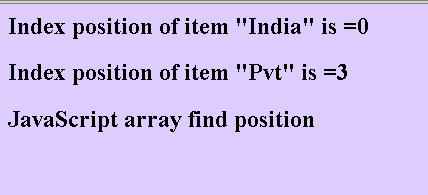Tutorials

# JavaScript array position

In this section of JavaScript array tutorial we have to find the position of the array items.

In this section of JavaScript array tutorial we have to find the position of the array items.

# JavaScript array position

In this section of JavaScript array tutorial we have to find the position of the array items. There is not any built in method in the JavaScript by which we can find the position of the array item therefore we have created a method getPosition() which takes two arguments: first is the array object and second is the item of which we have to find the position.

In this example of getting position we have traversed whole array for finding array element's position and where the specified item matched with the array elements it returns the index i.e the position of array item.

 function getPosition(arrayName,arrayItem) {   for(var i=0;i

Above lines of code defines a function getPosition(arrayName, arrayItem) for getting the index or position of the specified array item. Here is the full HTML example code of javascript_array_position.html as follows:

javascript_array_position.html

 JavaScript array position example

JavaScript array find position

Output of the example is as follows: